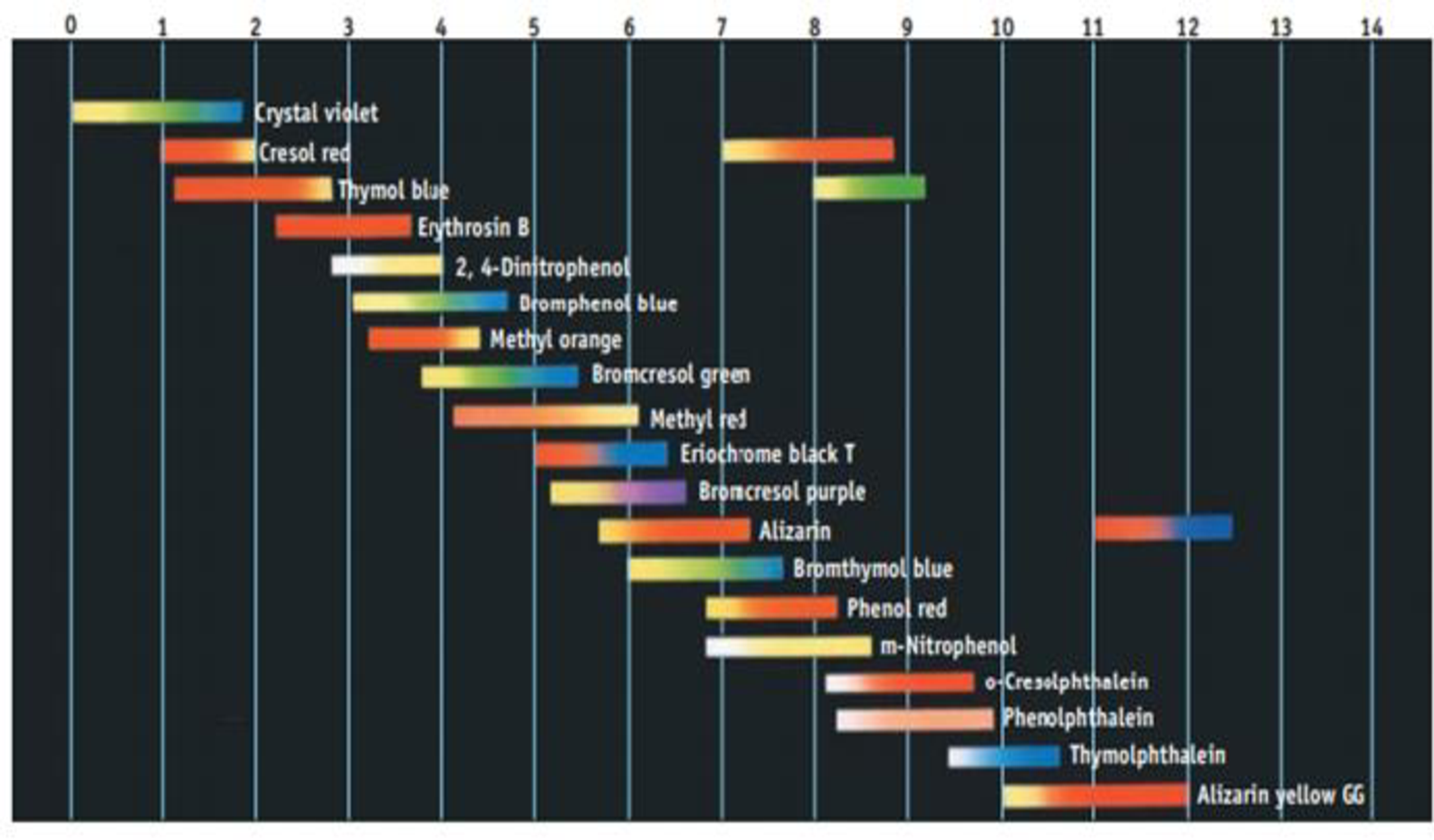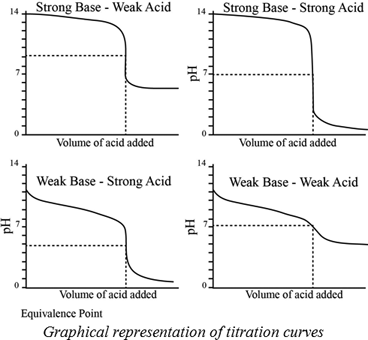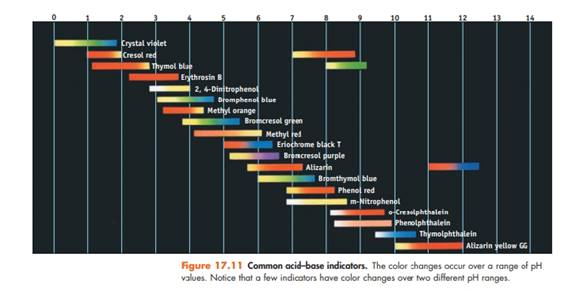Chapter 17, Problem 35PS

Chapter
Section
Textbook Problem

Using Figure 17.11, suggest an indicator to use in each of the following titrations: (a) The weak base pyridine is titrated with HCl. (b) Formic acid is titrated with NaOH. (c) Ethylenediamine, a weak diprotic base, is titrated with HCl.Figure 17.11 Common acid-base indicators. The color changes occur over a range of pH values. Notice that o few indicators hove color changes over two different pH ranges.

a)

Interpretation Introduction

Interpretation:

The weak base pyridine is titrated with HCl, an indicator used has to be determined.

Concept introduction:

A titration is one of the most useful ways of determining accurately the quantity of an acid, a base or some other substances in a mixture.

The pH at the equivalence point of a strong acid –strong base titration is 7.

A weak acid titrated with a strong base leads to a pH > 7 at the equivalence point.

A weak base titrated with a strong acid leads to pH < 7 at the equivalence point.Indicators:

A chemical substances which gives a visible change  in the titration.

Some important indicators are as follow.Explanation

Pyridine is a weak base and has a pkb= 8.82. Titrating a weak base with a strong acid HCl.

Therefore, the resultant solution pH will be less than the 7

b)

Interpretation Introduction

Interpretation:

Formic acid is titrated with NaOH , an indicator used has to be determined.

Concept introduction:

A titration is one of the most useful ways of determining accurately the quantity of an acid, a base or some other substances in a mixture.

The pH at the equivalence point of a strong acid –strong base titration is 7.

A weak acid titrated with a strong base leads to a pH > 7 at the equivalence point.

A weak base titrated with a strong acid leads to pH < 7 at the equivalence point.Indicators:

A chemical substances which gives a visible change  in the titration.

Some important indicators are as follow.c)

Interpretation Introduction

Interpretation:

Ethylene diamine, a weak diprotic base, is titrated with HCl, an indicator used has to be determined.

Concept introduction:

A titration is one of the most useful ways of determining accurately the quantity of an acid, a base or some other substances in a mixture.

The pH at the equivalence point of a strong acid –strong base titration is 7.

A weak acid titrated with a strong base leads to a pH > 7 at the equivalence point.

A weak base titrated with a strong acid leads to pH < 7 at the equivalence point.Indicators:

A chemical substances which gives a visible change  in the titration.

Some important indicators are as follow.Still sussing out bartleby?

Check out a sample textbook solution.

See a sample solution

The Solution to Your Study Problems

Bartleby provides explanations to thousands of textbook problems written by our experts, many with advanced degrees!

Get Started